# PSAT Math : How to add fractions

## Example Questions

### Example Question #1 : How To Add Fractions

Jesse has a large movie collection containing X movies. 1/3 of his movies are action movies, 3/5 of the remainder are comedies, and the rest are historical movies. How many historical movies does Jesse own?

(2/5)*X

(7/12)*X

(4/15)*X

(3/9)*X

(11/15)*X

(4/15)*X

Explanation:

1/3 of the movies are action movies. 3/5 of 2/3 of the movies are comedies, or (3/5)*(2/3), or 6/15. Combining the comedies and the action movies (1/3 or 5/15), we get 11/15 of the movies being either action or comedy. Thus, 4/15 of the movies remain and all of them have to be historical.

### Example Question #2 : How To Add Fractions

If x = 1/3 and y = 1/2, find the value of 2x + 3y.

13/6

2

5/6

6/5

1

13/6

Explanation:

Substitute the values of x and y into the given expression:

2(1/3) + 3(1/2)

= 2/3 + 3/2

= 4/6 + 9/6

= 13/6

### Example Question #3 : How To Add Fractions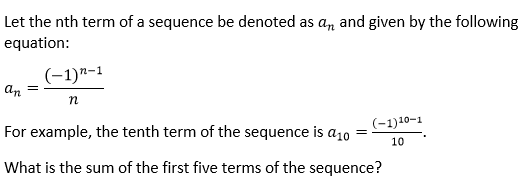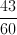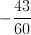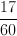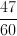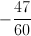Explanation: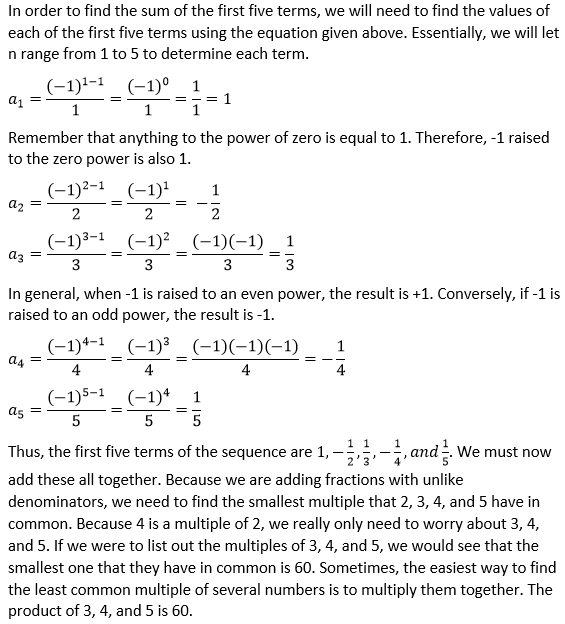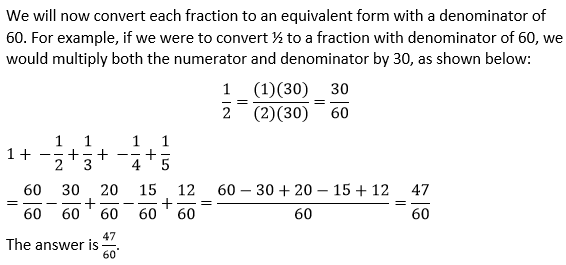### Example Question #7 : How To Add Fractions

Solve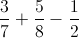.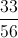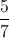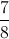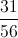Explanation:

Finding the common denominator yields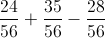. We can then evaluate leaving.

### Example Question #4 : How To Add Fractions

What is the solution, reduced to its simplest form, for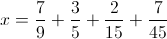?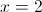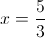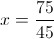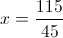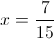Explanation: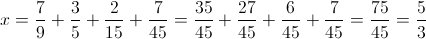### Example Question #131 : Fractions

What is the sum ofand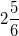?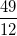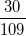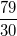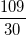Explanation:

We can begin by eliminating the obviously wrong answers. We know that the sum of the two fractions will be more than 1, so the answer choices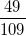andare out. Now, let's add the two fractions:

Begin by convertingto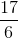.

Now find the common denominator ofand. The least common multiple of 5 and 6 is 30, so 30 is the common denominator. Now alter both fractions so that they use the common denominator: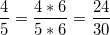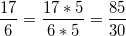Now we can easily add the two fractions together: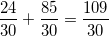The answer is.